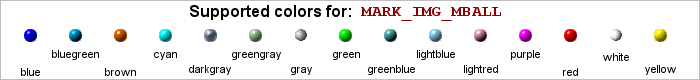## E.2. Built in image plot marks

Since the image based plot marks only supports some colors (since there has to be a unique image for each color) the following sections shows for each major image plot mark what colors are available. The image shows the natural size of the plot mark, i.e. the scale factor is =1. Only the round balls ar available natively in three different sizes. Even though it is possible to scale up all the images arbitrarily it will cause the images to become pixelated.

To use one of the built in image plot mark two parameters has to be given to the `SetType()` method. The basic class of plot mark and the wanted color. For example to use a green square as plot marks the following line would have to be added

 ```1 2 3 ``` ```mark->SetType(MARK_IMG_SQUARE,'green'); ?>```

If a non-supported color is specified an error will be thrown.

### E.2.1. Image plot mark: Balls

Figure E.1. Small sizeFigure E.2. Medium sizeFigure E.3. Large size### E.2.2. Image plot mark: Squares

Figure E.4. Standard size### E.2.3. Image plot mark: Diamonds

Figure E.5. Standard size### E.2.4. Image plot mark: Stars

Figure E.6. Standard size### E.2.5. Image plot mark: Bevels

Figure E.7. Standard size### E.2.6. Image plot mark: Pushpins

Figure E.8. Standard size